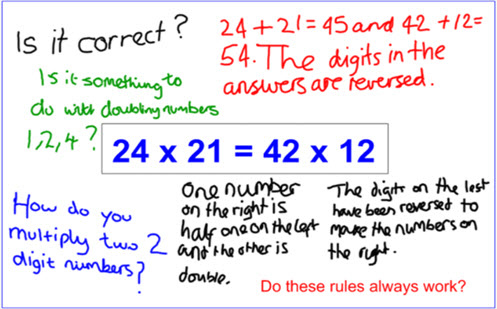Go to website

# Reversing digits

Year level(s) Year 5, Year 6
Audience Teacher
Teaching strategies and pedagogical approaches Collaborative learning, Differentiated teaching, Explicit teaching, Feedback, Mathematics investigation
Keywords multiply, double, halve

## Curriculum alignment

Curriculum connections Critical and creative thinking, Numeracy
Strand and focus Number, Apply understanding, Build understanding
Topics Multiples, factors and powers
AC: Mathematics (V9.0) content descriptions
AC9M5N06
Solve problems involving multiplication of larger numbers by one- or two-digit numbers, choosing efficient calculation strategies and using digital tools where appropriate; check the reasonableness of answers

AC9M6N09
Use mathematical modelling to solve practical problems, involving rational numbers and percentages, including in financial contexts; formulate the problems, choosing operations and efficient calculation strategies, and using digital tools where appropriate; interpret and communicate solutions in terms of the situation, justifying the choices made

Multiplicative strategies (P7, P8)
Understanding money (P8)